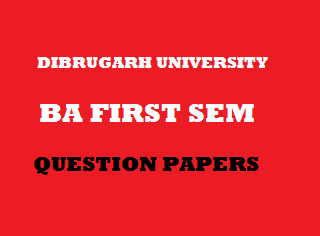## Friday, January 04, 20192012
(November)
ECONOMICS
(General)
Course: 101
(Microeconomic Theory)
Full Marks: 80
Pass Marks: 32
Time: 3 hours
The figures in the margin indicate full marks for the questions

1. Answer the following questions/Fill in the blanks: 1x8=8
1. What do you mean by micro statics?
2. Who is the exponent of the theory of monopolistic competition?
3. In case of unit elastic demand when the price of a commodity decreases by 7%, the demand for its increases by ____.
4. What is the basic objective of a firm?
5. Define rent.
6. What is money cost?
7. Give the meaning of consumer surplus.
8. According to ____, the rate of interest depends on demand for and supply of money.
2. Write short notes on any four (within 150 words each) of the following: 4x4=16
1. Comparative micro statics.
2. Indifference curve.
3. Relationship among total cost, total fixed cost and total variable cost.
4. Normal Profit.
5. Quasi rent.
Answer the following questions (within 500 words each):
3. (a) Explain briefly the subject matter of microeconomics. 10
Or
4. (a) What is marginal utility? Explain the law of diminishing marginal utility with diagram. 2+8=10
Or
(b) Show the difference between point elasticity and are elasticity of demand. 10
5. (a) Explain with diagram, the concepts of average production cost, average fixed cost, average variable cost and marginal cost. 3x4=12
Or
(b) What do you mean by average revenue and marginal revenue? Why does a firm attain equilibrium when its marginal cost equals to marginal revenue? 2+10=12
6. (a) Discuss the price and output determination under monopolistic competition. 12
Or
(b) Explain the difference between perfectly competitive market and monopoly market. 12
7. (a) Explain briefly the marginal productivity theory of distribution. 12
Or
(b) Explain the risk bearing theory of profit. Mention two basic differences of this theory with that of uncertainty bearing theory of profit. 10+2=12

***